# lucene系列(六)索引格式之fdt文件

## 前言

fdt 文件，以正排的方式，存储了 field 的原始真实数据。也就是说，你添加到所有中的所有 field 内容。都会存储在此文件中。

## .fdt 文件整体结构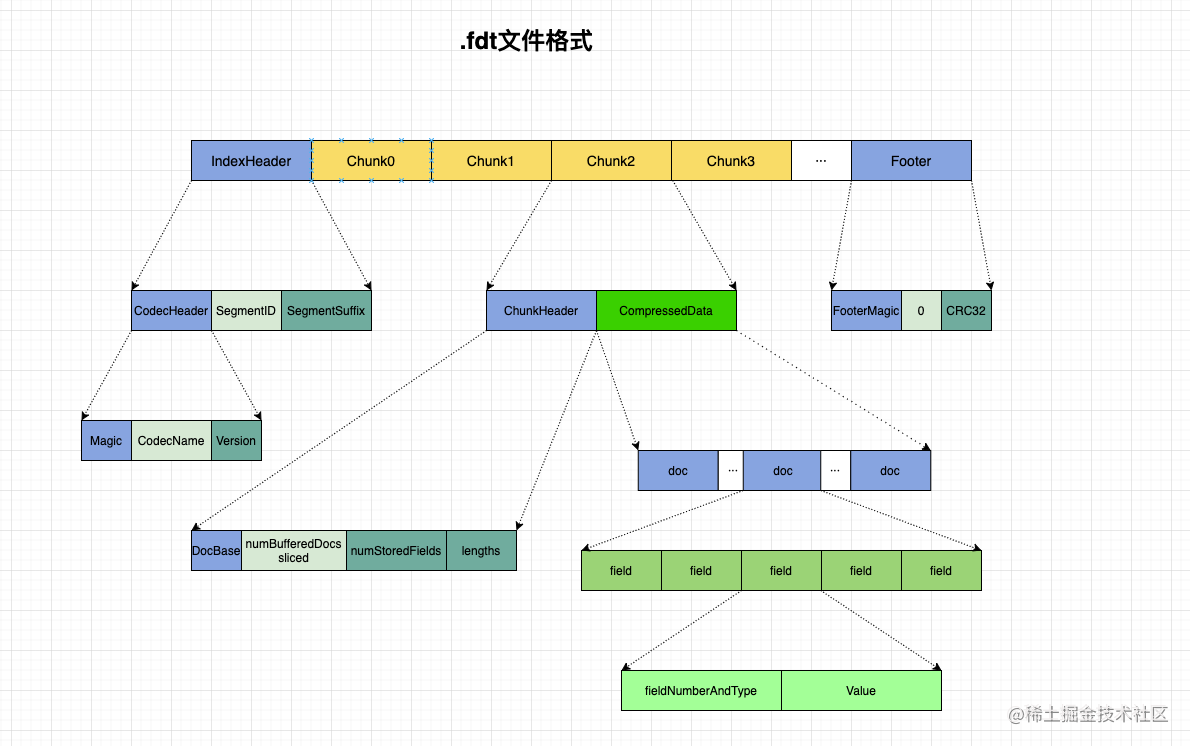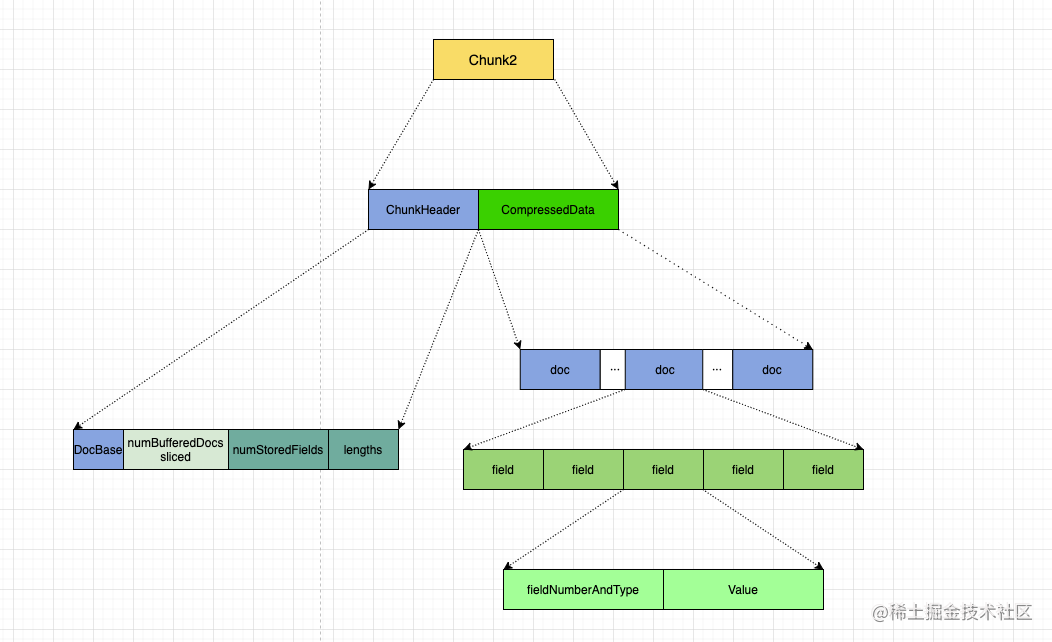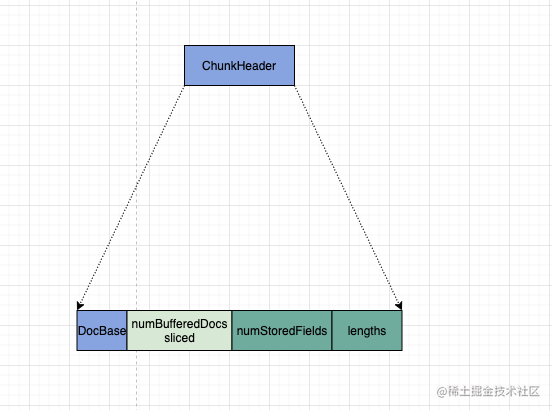• docBase : 当前 chunk 里的第一个 docID.
• numBufferedDocs << | slice . 当前块里面缓冲了多少个 doc, 可以根据 docBase 及 num 来算出每一个 docId. 还以 bit 的方式存储了当前 chunk 是否分片。
• 存储每个文档有多少个 field.　（数组）
• 存储每个文档的 field 信息长度（字节长度） （数组）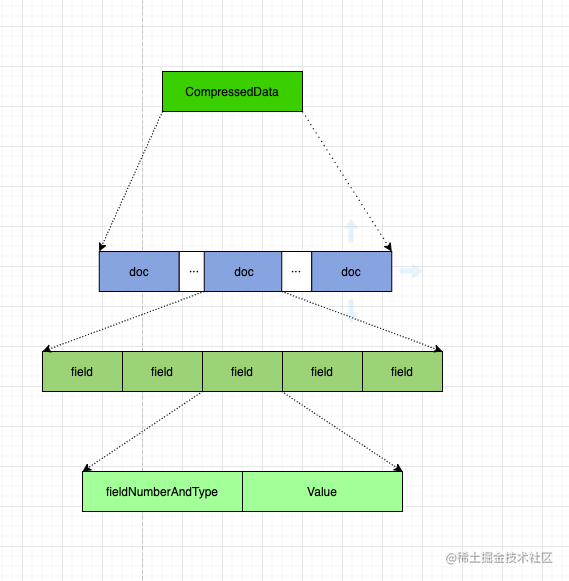field 信息中，存储了：

1. FieldNumberAndType: field 的编号及类型
2. Value: 实际的值，根据不同类型 (int,long,string,bytes 等）, 存储方法不同。

## 写入代码分析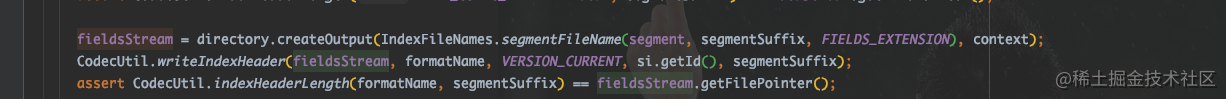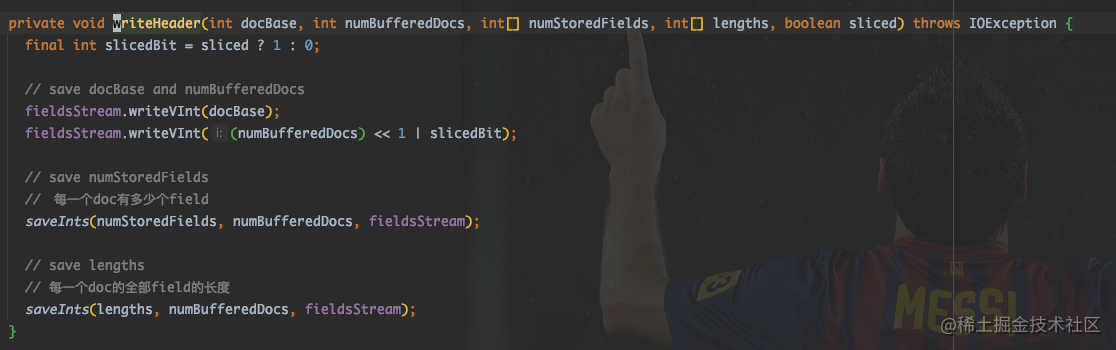``````  /**
* 写了什么？
* １．编号及类型
* 2. 内容
*    2.1 如果是基本类型，直接存储
*    2.2 如果是 bytes, 写长度和内容
*    2.3 如果是 string, 先写长度，然后写内容
*/
@Override
public void writeField(FieldInfo info, IndexableField field)
throws IOException {

// 计数+1
++numStoredFieldsInDoc;

int bits = 0;
final BytesRef bytes;
final String string;

Number number = field.numericValue();
if (number != null) {
if (number instanceof Byte || number instanceof Short || number instanceof Integer) {
bits = NUMERIC_INT;
} else if (number instanceof Long) {
bits = NUMERIC_LONG;
} else if (number instanceof Float) {
bits = NUMERIC_FLOAT;
} else if (number instanceof Double) {
bits = NUMERIC_DOUBLE;
} else {
throw new IllegalArgumentException("cannot store numeric type " + number.getClass());
}
string = null;
bytes = null;
} else {
bytes = field.binaryValue();
if (bytes != null) {
bits = BYTE_ARR;
string = null;
} else {
bits = STRING;
string = field.stringValue();
if (string == null) {
throw new IllegalArgumentException("field " + field.name() + " is stored but does not have binaryValue, stringValue nor numericValue");
}
}
}

// 存储了 field 的内部编号，以及当前 field 的类型，是四种数字呢，还是字符串，还是二进制串。
// number , 一个 int, 右边的 3 位是类型，左边的是编号
final long infoAndBits = (((long) info.number) << TYPE_BITS) | bits;
bufferedDocs.writeVLong(infoAndBits);

if (bytes != null) {
bufferedDocs.writeVInt(bytes.length);
bufferedDocs.writeBytes(bytes.bytes, bytes.offset, bytes.length);
} else if (string != null) {
bufferedDocs.writeString(string);
} else {
if (number instanceof Byte || number instanceof Short || number instanceof Integer) {
bufferedDocs.writeZInt(number.intValue());
} else if (number instanceof Long) {
writeTLong(bufferedDocs, number.longValue());
} else if (number instanceof Float) {
writeZFloat(bufferedDocs, number.floatValue());
} else if (number instanceof Double) {
writeZDouble(bufferedDocs, number.doubleValue());
} else {
throw new AssertionError("Cannot get here");
}
}
}